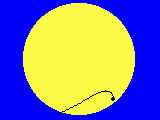Essen, horizontal view

# Transit of Venus, June 8th, 2004Essen, equatorial view

## Project 3: Determining the Radius of the Earth

To be able to derive an own measure of the Astronomical Unit from the self determined value of the Sun's parallax by the following equation (see the related paper (in German or in English))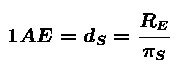you need to determine the radius RE of the Earth.

For this reason, you may contact other participants of our project who are suitably located with respect to your own site.

Because there are other collaborative projects which have been executed in the past and the material of which can still be found in the internet (see, for instance, the Erathostenes project and its final report) the basic idea and some methods of measurement are described here only shortly.

### Basic Idea

The Earth's radius can be calculated if, for some path on the surface of known length s, the central angle alpha can be measured. But how is it possible to measure an angle at the Earth's center? It was the ingenious idea of Erathostenes to use the fact that the sunlight enters different sites on Earth in parallel direction and to make use of the methods of "geo-metry" in order to determine the unreachable angle in the center by measuring two angles z1 and z2 on the Earth's surface.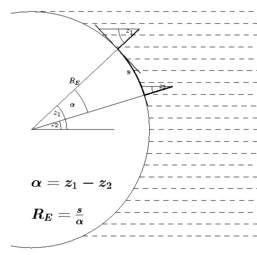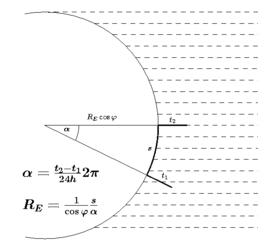Plane containing the common circle of longitude of the both observers, the center of the Earth and the Sun. The axis of rotation lie in the plane of paper. Plane containing only the common circle of latitude of the both observers. The axis of rotation is perpendicular to plane of paper.

### Methods of Measurement

1. Two observers of (about) the same longitude

For these observers, it is not necessary to use a clock. Both of them measure the length lS of the shortest shadow of a vertical pole of length l. In that moment it is local noon, that means the Sun culminates. Than, the angles zi can easily be calculated from the ratio lS/l.

The central angle and the Earth's radius can than be calculated as shown in the left picture above.

2. Two observers of (about) the same latitude

For these observers, it is crucial to have synchronized clocks because they must exactly measure the time at which the length of the shadow of a vertical pole is at its minimum. As, around the time of culmination, the length of the shadow is changing only slightly it is better to determine the time of the shortest shadow by the method connoted in the picture below: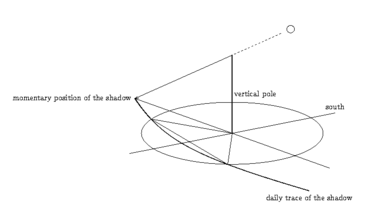1. Put the vertical pole in the center of a suitably sized circle.
2. Measure the two times at which the length of the shadow equals exactly the radius of the circle.
3. Than, the Sun has culminated exactly at the central time of these two moments.

The central angle which is, in this case, located not at the Earth's center but at the axis of rotation, and the Earth's radius can than be calculated as shown in the right picture above. The geographical latitude must be known.

In both cases, the distance between the observers along the Earth's surface must be known. Usually, it will be taken, for instance, from a map. Nevertheless, it would be more satisfying to determine that distance by an own trip but that is possible only for short distances. But in that case, one has to measure the length of the shadow very precisely.

If you use a pin hole instead of the tip of a stick it is possible to reduce the error to about 0.1 degree. Than, you may be able to measure the distance by bike: The angle belonging to a trip of 30 kilometers is about 0.25 degree.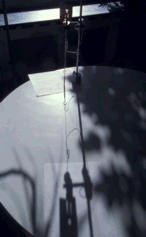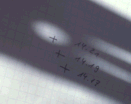Pinhole and pendulum serve as a vertical pole. The pinhole generates an elliptic picture of the Sun whose center can be located very precisely.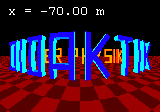Prof. Dr. Udo Backhaus
last modification:  July 28th, 2008